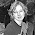## miércoles, 24 de octubre de 2012

### SEAL-Stream Cipher

A more secure SEAL was designed in 1992 by Rogaway and Coppersmith, speci cally for the purpose of obtaining a software effi cient stream cipher. SEAL is still the fastest steam cipher for software implementations on contemporary PC's, with "C" implementations running at 5 cycle/byte on common PC's (and 3.5 cycle/byte on some RISC workstations).

The design of SEAL shares many similarities with the design of common block ciphers. It is built around a repeating round function, which provides the "cryptographic strength" of the cipher.

Algorithm optimized for 32-bit processors.
• Jogging requires eight 32-bit registers and a cache a few kilobytes
• Through a seemingly slow operation, SEAL preprocesses the operation of keys in a set of tables.
• Then these tables are used to increase the speed of encryption and description
SEAL is not a traditional flow cryptosystem, this is a pseudo functions family, is a family of functions pseudo - random.

ITS OPERATION
Its operation is based on a initial process in which are calculated values for some tables from the key, so that proper encryption can be carried out in a way really fast. Unfortunately, it is also subject to patent algorithm.
Given a key 160-bit, k and a number n of 32-bit
• SEAL extends a string of L n k bits (n)
• L can take any value less than 64 kilobytes
• Is supposed to SEAL takes advantage of the property of k is selected randomly
• Then k (n) may be that not distinguished computationally a random function of L 'n' bits
A very useful feature of this algorithm is that it is not based on a linear system of
generation, but that of a family of pseudorandom functions, in such a way that any part of the sequence can be calculated providing only a whole number n of 32-bit.
With a cryptosystem as SEAL
• You can access any bits of the generated key
Use:
•  Make a hard drive
• Encrypt by and every sectors of 512 bytes
• With SEAL is possible to encrypt the contents of the sector n, with an XOR of the content with the key k (n).

SEAL is based on the use of the SHA algorithm (see section 13.1.4 on) to generate the tables
that it uses internally. In fact, there are two versions of the algorithm, the 1.0 and 2.0, that differ precisely in that the first employs SHA and the second its on revised version, SHA-1.

Algorithm controlled by three tables derived keys: R, y, S, and T
• Preprocessing maps the key k, to these values using a procedure based on SHA
Also used four 32-bit registers: A, B, C and D
• Initial values are determined by n and R and T tables
• These records are modified through several iterations, each one involves 8 turns iterations, each one involves 8 laps.
• In each iteration 9 bits of a register are used to index the table T.
• Content table gives a xor with the contents of a second record A, B, C and D.
• First record is travel (shifted) 9 positions.
In some iterations the second record is modified amended through an xor with the first now travel record
• After 8 iterations of this, A, B, C and D are added to the stream of the key
• The iteration is completed by adding to A and C additional values depending on n n1 n2 additional depending on n, n1, n2, n3 and n4 values.
• Exactly which depends on the parity of the number of iteration.
Function Seal

Here compares the efficiency of each stream cipher Stream cipher

Referencia:

#### 1 comentario:

1.En lo de ejemplo y vulnerabilidades pudieras haber mejorado. Van 5 pts.